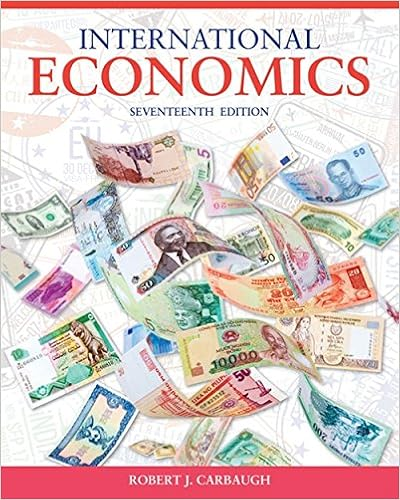# Draw the tree for a put option on 20000 with a strike

• Test Prep
• 75
• 92% (93) 86 out of 93 people found this document helpful

This preview shows page 56 - 60 out of 75 pages.

##### We have textbook solutions for you!
The document you are viewing contains questions related to this textbook.The document you are viewing contains questions related to this textbook.
Chapter 11 / Exercise 7
International Economics
CarbaughExpert Verified
58. Draw the tree for a put option on \$20,000 with a strike price of £10,000. The current exchange rate is £1.00 = \$2.00 and in one period the dollar value of the pound will either double or be cut in half. The current interest rates are i\$= 3% and are i£= 2%. A. B.C. None of the aboveTopic: Binomial Option-Pricing Model7-56
##### We have textbook solutions for you!
The document you are viewing contains questions related to this textbook.The document you are viewing contains questions related to this textbook.
Chapter 11 / Exercise 7
International Economics
CarbaughExpert Verified
Chapter 07 - Futures and Options on Foreign Exchange59. Draw the tree for a call option on \$20,000 with a strike price of £10,000. The current exchange rate is £1.00 = \$2.00 and in one period the dollar value of the pound will either double or be cut in half. The current interest rates are i\$= 3% and are i£= 2%. A. B.C. None of the aboveTopic: Binomial Option-Pricing Model7-57
Chapter 07 - Futures and Options on Foreign Exchange60. Find the hedge ratio for a put option on \$15,000 with a strike price of €10,000. In one period the exchange rate (currently S(\$/€) = \$1.50/€) can increase by 60% or decrease by 37.5% (i.e.u= 1.6 and d= 0.625). A.-15/49B. 5/13C. 3/2D. 15/49Topic: Binomial Option-Pricing Model61. Find the hedge ratio for a put option on €10,000 with a strike price of \$15,000. In one period the exchange rate (currently S(\$/€) = \$1.50/€) can increase by 60% or decrease by 37.5% (i.e. u= 1.6 and d= 0.625). A. -15/49B. 8/13C.-5/13D. 15/49Topic: Binomial Option-Pricing Model62. Find the dollar value today of a 1-period at-the-money calloption on €10,000. The spot exchange rate is €1.00 = \$1.25. In the next period, the euro can increase in dollar value to \$2.00 or fall to \$1.00. The interest rate in dollars is i\$= 27.50%; the interest rate in euro is i=2%. A.\$3,308.82B. \$0C. \$3,294.12D. \$4,218.75Topic: Binomial Option-Pricing Model7-58
Chapter 07 - Futures and Options on Foreign Exchange63. Suppose that you have written a call option on €10,000 with a strike price in dollars. Suppose further that the hedge ratio is ½. Which of the following would be an appropriate hedge for a short position in this call option? A. Buy €10,000 today at today's spot exchange rate.B. Buy €5,000 today at today's spot exchange rate.C. Agree to buy €5,000 at the maturity of the option at the forward exchange rate for the maturity of the option that prevails today (i.e., go long in a forward contract on €5,000).D. Buy the present value of €5,000 discounted at i€ for the maturity of the option.E.Both c) and d) would work.F. None of the aboveTopic: Binomial Option-Pricing Model64. Find the value of a one-year put option on \$15,000 with a strike price of €10,000. In one year the exchange rate (currently S0(\$/€) = \$1.50/€) can increase by 60% or decrease by 37.5% (i.e. u= 1.6 and d= 0.625). The current one-year interest rate in the U.S. is i\$= 4% andthe current one-year interest rate in the euro zone is i= 4%. A. €1,525.52B. \$3,328.40C. \$4,992.60D.€2,218.94E. None of the aboveTopic: Binomial Option-Pricing Model
•••